# 介绍

StarSpace是一种神经嵌入模型（neural embedding model ），可以广泛解决多种问题：

• 文本分类，或者其它标注任务，比如：语义分类
• 实体排序，比如：给定一个query，对web文档进行排序
• 基于CF的推荐，例如：文档、音乐、或视频推荐
• 基于内容的推荐，其中，内容通过离散特征（例如：词）定义
• 图谱嵌入（mebedding graphs），例如，Freebase这样的多关系图谱
• 学习词、句、文档的embeddings

# 模型

StarSpace模型由学习实体（learning entities）组成，每个通过一个离散特征（bag-of-features）的集合描述。一个像（文档、句子）这样的实体可以通过bag-of-words或n-grams进行描述，一个像user这样的实体可以通过bag-of-documents、movies、items的方式进行描述。重要的是，StarSpace模型可以自由比较不同类型的实体（entities）。例如，一个用户实体可以与一个item实体、或者一个文档实体(推荐)、一个文带标签实体的文档实体等进行比较。这可以通过学习来完成，并将它们嵌入到相同的空间中，这样的比较才有意义————通过根据各自的metric进行最优化。

$\sum_{(a,b)\inE^+, b^- \in E^-} L^{batch} (sim(a,b), sim(a,b_1^-), ..., sim(a,b_k^-))$

• 正实体对 positive entity pairs (a,b)的生成器来自于集合$E^+$。这是任务非独立的，将会在下面描述。
• 负实体$b_i^-$的生成器来自于集合$E^-$。我们使用一个k-negative sampling策略（Mikolov 2013）来为每个batch update选择k个这样的负样本对。我们会从在该实体集内随机选择，它们可能出现在相似函数的第二个参数上（例如：对于文本标注任务, a是文档，b是标签，因此我们可以从labels集合上抽样$b^-$）。k的因素分析将在第4部分讨论。
• 相似函数$sim(\cdot, \cdot)$。在我们的txxik，我们的实现有：cosine相似度和内积，可以通过一个参数进行选定。总之，对于较少数目的label features（比如：分类），它们的工作机制很像；对于更大数目（比如：句子或文档相似度）时，cosine更好。
• loss function为 $L_{batch}$，它比较了positive pair (a,b)，negative pairs(a, b_i^-)，其中i=1,…, k. 我们也实现了两个概率：margin ranking loss（例如：$max(0, \mu-sim(a,b))$，其中$\mu$是margin参数），negative loss loss of softmax。所有的实验都使用前者，因为表现更好。

$Multiclass分类（例如：文本分类）$：positive pair generator直接来自于一个标注数据训练集，(a,b) pairs，其中，a是文档（bags-of-words），b是labels（单个features）。负实体($b^-$)从可能的labels集合中被抽样。

$Multilabel Classification$: 在该case中，每个文档a可以具有多个正标签，其中之一在每个SGD step中从b中抽样，来实现multilabel分类。

$基于CF的推荐$：训练数据包含了：一个用户集合，其中每个用户通过bag-of-items进行描述（字典里的唯一特征）。positive pair生成器会选择一个user，选择a作为该user ID的唯一单个特征，以及单个item b。负实体$b^-$从该可能的items中进行抽样。

• (i) a由bag-of-features：h和r 组成，其中b只包含t；
• (ii) a由h组成，b包含了r和t.

# Deep AutoEncoders for CF介绍

NVidia在2017年提出了《Training Deep AutoEncoders for Collaborative Filtering》：

# 1.介绍

Amazon, Netflix和Soptify均使用推荐系统给用户推荐items。推荐系统分为两类：基于上下文的推荐(context-based)，基于个性化的推荐(personized)。

# 2.模型

• a) 使用SELU（scaled exponential linear units）
• b) 使用较高的dropout
• c) 在训练期间使用迭代型output re-feeding

• encoder encode(x): $R^n \rightarrow R^d$
• decoder(z): $R^d \rightarrow R^n$

autoencoder的目标是获取数据的d维数据，以确保在x和$f(x)=decode(encode(x))$间的error measure是最小化的。图1描述了典型的4-layer autoencoder网络。如果在encoding阶段将噪声添加到该数据中，该autoencoder会被称为de-noising。Autoencoder是一个很好的降唯工具，可以被认为是一种严格泛化的PCA。一个没有非线性激活函数、只有“code” layer的autoencoder，可以在encoder中学习PCA转换，以MSE loss进行最优化。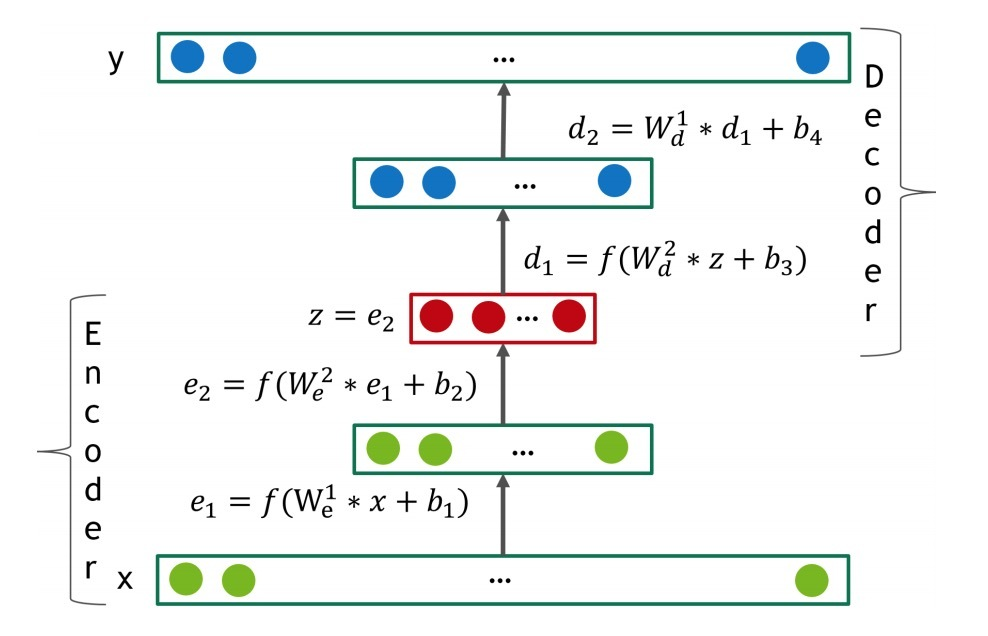## 2.1 Loss function

$MMSE = \frac{m_i * (r_i - y_i)^2} {\sum_{i=0}^{i=n} m_i}$

…(1)

• 如果$r \neq 0$则$m_i=1$
• 否则为$m_i=0$

## 2.2 Dense re-feeding

$f(x)_i = x_i， \forall i: x_i \neq 0$，

• 1.给定稀疏x，使用等式(1)来计算dense f(x)和loss
• 2.计算梯度、执行权重更新(backward pass)
• 3.将f(x)看成是一个新的样本，计算f(f(x))。现在f(x)和f(f(x))是dense的，来自等式(1)的loss上所有m项都是非零的（第二个forward pass）
• 4.计算梯度、执行weight更新（第二个backward pass）

# 3.实验和结果

## 3.2 激活函数类型的影响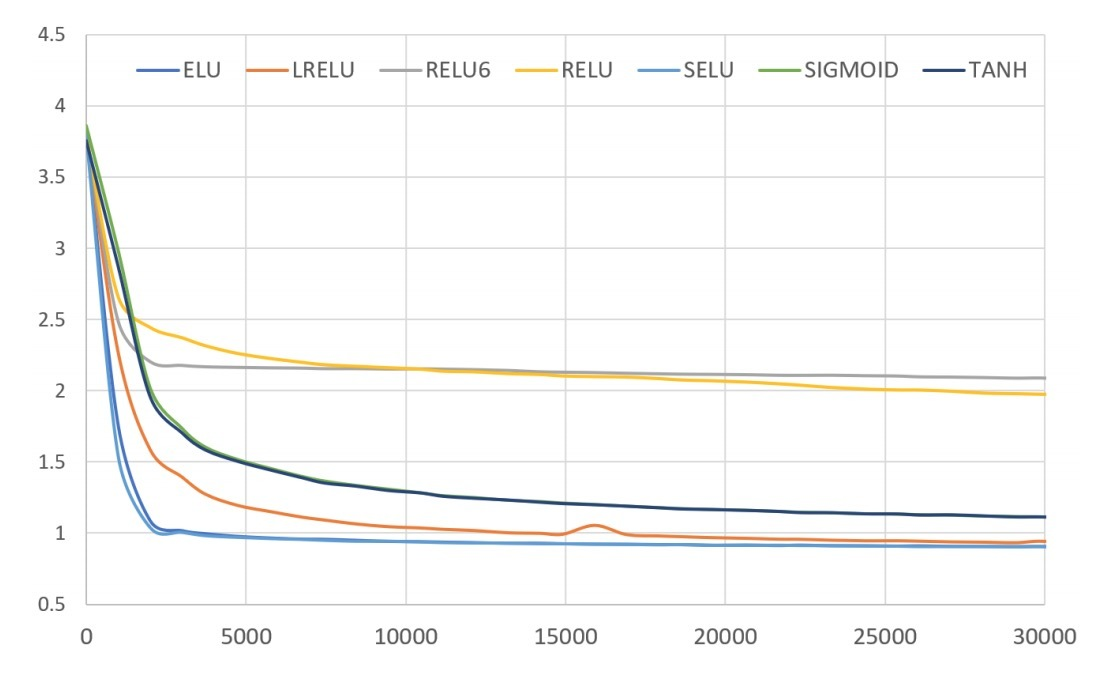• a) non-zero negative part
• b) unbounded positive part

## 3.3 overfitting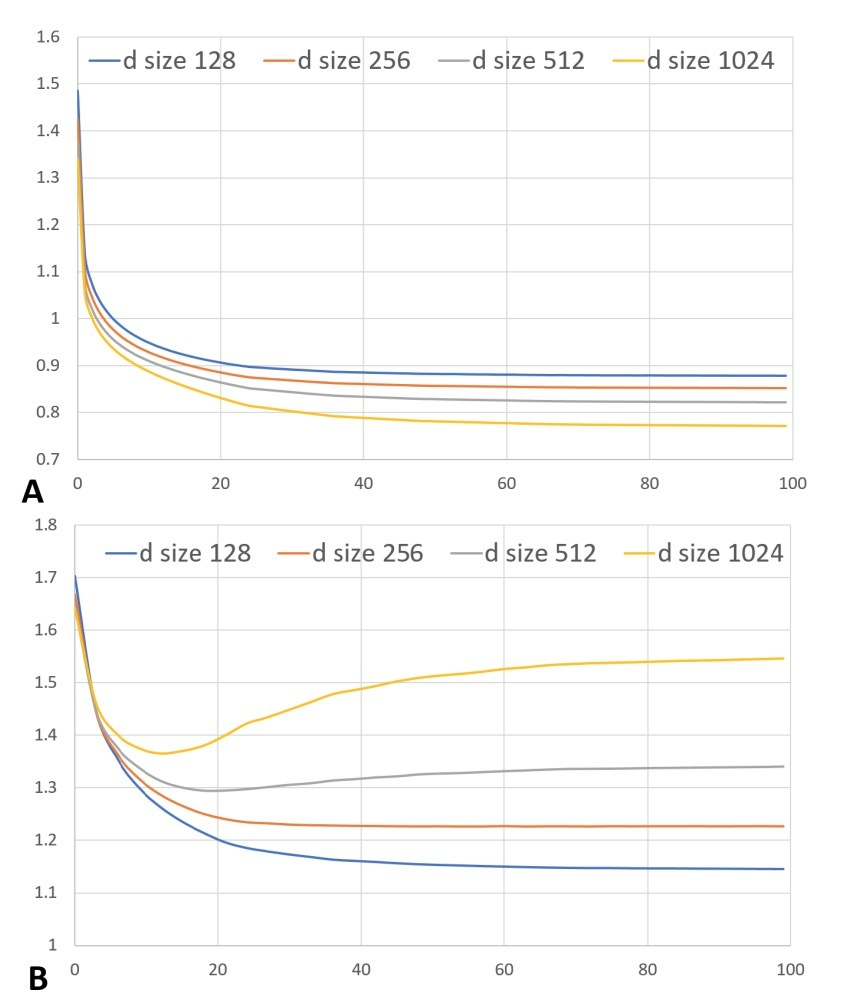## 3.5 Dropout

n, 512, 512, 1024, 512, 512, n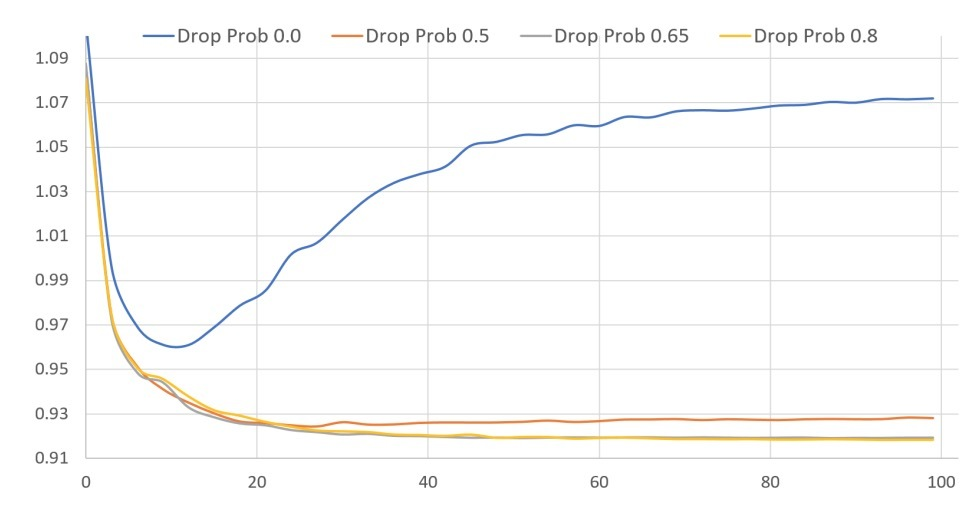## 3.6 Dense re-feeding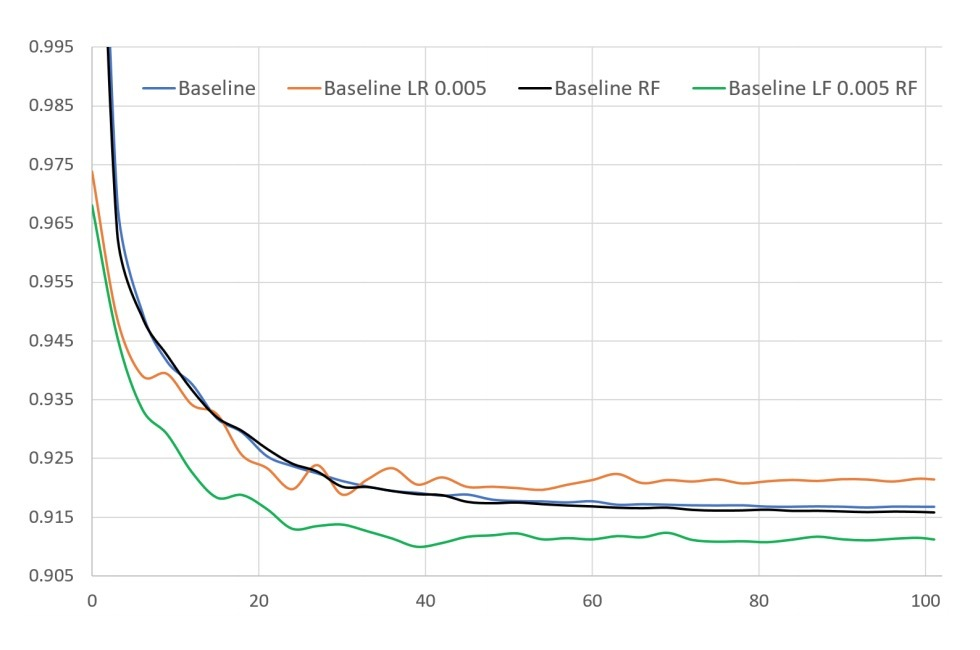## 3.7 与其它方法的比较

“Netflix 3 months”的训练数据比”Netflix full”要小7倍，也就是说，在模型效果上差些并不吃惊（0.9373 vs. 0.09099）。事实上，在”Netflix full”上效果最好的模型会在该集合上over-fits，我们必须减小模型复杂度（见表4）

# 参考

https://arxiv.org/pdf/1708.01715.pdf

# CRNN介绍

CRNN( Convolutional Recurrent Neural Network)由华科白翔等人提出。

# 介绍

crnn主要关注CV中的一个经典难题：基于图片的序列识别。现实世界中，一大群视频对象，比如场景文字（scene text）、手写、音阶符，以序列方式出现。不同于通用目标识别，识别这样的序列对象通常需要系统去预测一串对象labels，而非单个label。因而，识别这样的目标很自然地转化成一个序列识别问题。序列化目标的另一个独特属性是，它们的长度变化很大。例如，英文词汇可以包含2个字符“OK”，也可以包含15个符如“congratulations”。因而，大多数流行的deep模型（比如DCNN）不能直接应用于序列预测，因为DCNN模型经常在固定维度的inputs和outputs上操作，不能产生变长的label sequence

RNN模型是Deep模型的另一分支，主要用于处理序列。RNN的一个好处是，它不需要知道在训练和测试时一个序列目标图片中每个元素的位置。然而，需要有一个预处理step来将一个输入目标图片转化成一个图片特征序列。例如，Graves et al.从手写文本中抽取一个几何集合 或者 图片特征，而paper将word images转化成顺序化的HOG特征。这些预处理step与pipeline中的子任何组件相互独立，因而，基于RNN的系统不能以一种end-to-end的方法地直接被训练和优化

• 1) 它可以直接从sequence labels（例如：words）中学习，无需详细注解（例如：characters）
• 2) 在从图片数据中直接学习有信息表示时，它具有DCNN的相同特性，即不用手工特征，也不用预处理steps（包括：二值化/分割，成分定位等）
• 3) 它具有RNN的相同属性，可以产生一个labels序列
• 4) 它不限制序列化对象的长度，在训练和测试阶段只需要高度归一化
• 5) 它在场景文字识别上，比之前的方法要好
• 6) 比起标准的DCNN，它包含了更少的参数，消耗更少的存储空间

# 2.网络结构

CRNN的网络结构如图1所示，自底向上，包含了三个组成部分：

• 卷积层（conv layers）
• 递归层（recurrent layers）
• 一个合成层（transcription layer）## 2.1 特征序列抽取## 2.2 Sequence Labeling

• 首先，RNN具有很强的能力来捕获在序列中的上下文信息。对于基于图片的序列识别使用上下文信息，比将每个符号单独对待的方式更稳定更有用。将场景文本识别看成一个示例，宽字符可能需要许多个连续帧才能完整描述（如图2所示）。另外，当观察它们的上下文时，一些模糊的字符很容易区分；例如，很容易识别“il”，通过区别该字符高度而非单个字符单独识别。
• 第二，RNN可以对error微分进行反向传播至它的输入（例如：conv layer），从而允许我们在同一网络中对recurrent layers和conv layers进行jointly training。
• 第三，RNN能在特有长度的序列上操作，从头到尾进行遍历。

LSTM是有向的，它只使用过去的上下文。然而，在基于图片的序列中，两个方向的上下文都是有用的。因而，我们使用了两个LSTMs来组成双向LSTM：一个向前，一个向后。另外，多个bi-LSTMs可以进行stack，产生一个如图3.b所示的deep bi-LSTM。deep结构比shallow结构允许更高级的抽象，在语音识别上可以达到极大的效果提升。### 2.3.1 label序列的概率

$p(l|y) = \sum_{\pi : B(\pi) = 1} p(\pi | y)$ …(1)

## 2.3.3 Lexicon-based transcription

$I^{*} = arg max_{I \in N_{\delta}(I')} p(l | y)$…(2)

## 2.4 网络训练

$O = - \sum_{I_i, l_i \in X} log p(l_i | y_i)$ …(3)

# 3.试验

## 3.1 Datasets

• ICDAR 2003(IC03)：该测试数据集包含了带标注文本边框的251张场景图片。根据paper，我们忽略了那些包含非字母数字字符、以及那些小于三个字符的图片，获得的测试集包含了860个裁减过的文本图片。每张测试图片与一个50-words的词典相关联（由paper定义）。一个完整的词典可以通过组合所有单张图片的词典来构成。另外，我们使用了一个50k个词的词典，它包含了在Hunspell spell-checking dictionary字典中的所有词。
• ICDAR 2013 (IC13)：测试数据集继承了IC03上的大多数数据，它包含了1015张ground truth的裁减word images。
• IIIT 5k-word (IIIT5k)：包含了3000张来自互联网上的word test images。每张图都与一个50词的词典和1k-words词典相关。
• Street View Text (SVT)：该测试数据集包含了来自Google Street View的249张街景门牌图片。从它们中裁减出647张word images。每张word images具有一个由paper定义的50 words的词典。

## 3.2 实现细节conv layers基于一个VGG-VeryDeep架构（paper）。为了让它更适合识别英文字符，会做一定调整。在第3和第4个max-pooling layers中，我们采用了1x2 的rectangular pooling window来替代常见的squared方法。该tweak yields的feature maps具有更大的宽度，更长的feature序列。例如，一张包含了10字符的图片，通常size=100x32, 其中一个feature sequence可以生成25帧。该长度超过了最大英文词汇的长度。在那之上，rectangluar pooling windows会生成rectangular receptive fields（如图2），它有益于识别具有narrow shapes的一些字符，比如’i’和’l’。

# 2.前置

## 2.1 使用隐式数据进行学习

$y_{ui} = \begin{cases} 1, \\ 0 \end{cases}$

…(1)

$y_{ui}$为1表示：user u和item i间有交互；然而，它并不意味着u实际会喜欢i。相似的，该值为0也并不表示用户u不喜欢item i。对隐式数据的学习存在着许多挑战，因为它存在许多关于用户偏好的噪声数据。而已观察条目至少反映了用户在这些items上的兴趣，未观察条目可能只是缺失数据（missing data），它天然缺少负反馈

• 作为在显式反馈上的一个天然扩展，pointwise learning方法通常会使用一个回归框架，对$\hat{y}_{ui}$与它的目标值$y_{ui}$间的平方loss（squared loss）进行最小化。为了处理缺失的negative数据，会将所有未观察条目当成是负反馈，或者从未观察条目中进行抽样。
• pairwise learning的思想是：已观察条目应比未观察条目排序更靠前。pairwise learning会最大化已观察条目$\hat{y}_{ui}$与未观察条目$\hat{y}_{uj}$间的间隔。

## 2.2 矩阵分解（MF）

MF会分别将每个user和item使用一个关于隐特征的real-valued vector进行关联。假设：

• $p_u$和$q_i$表示user u和item i的隐向量

MF会将对$y_{ui}$的估计表示成$p_u$和$q_i$的内积：

$\hat{y}_{ui}=f(u,i|p_u,q_i) = p_u^T q_i = \sum\limits_{k=1}^{K} p_{uk} q_{ik}$

…(2)

MF会建模关于user和item隐因子的two-way interaction，假设隐空间的每个维度相互独立，并使用相同的权重进行线性组合。这样，MF可以被看成是一个关于隐因子的线性模型。

• 首先，由于MF会将users和items映射到相同的隐空间上，两个用户间的相似度可以通过一个内积、或者隐向量间夹角的cosine来进行度量。
• 第二，不失一般性，我们可以使用jaccard系数作为MF所需要去恢复的两个用户间的ground truth相似度。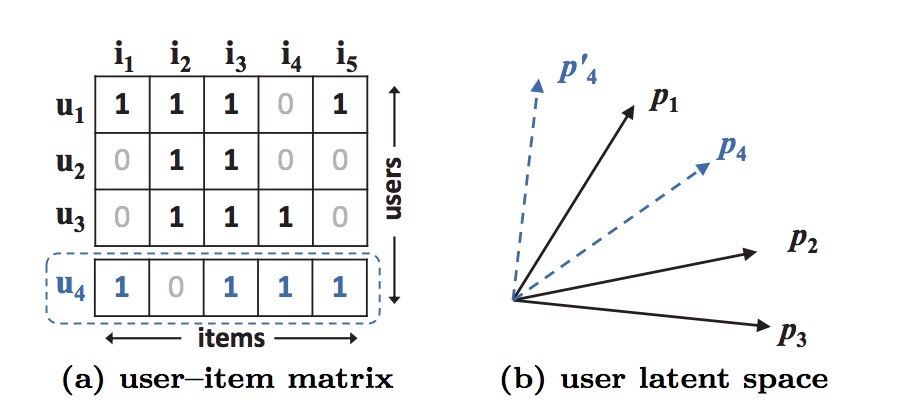# 3. neural CF

## 3.1 通用框架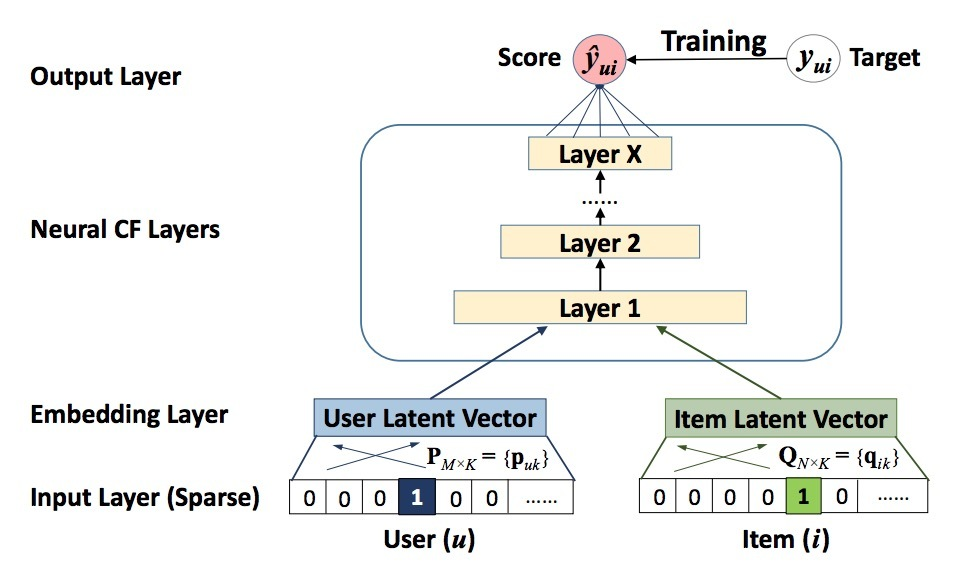$\hat{y}_{ui} = f(P^T v_u^U, Q^T v_i^I | P, Q, \theta_f)$

…(3)

$f(P^T v_u^U, Q^T v_i^I) = \phi_{out} (\phi_X (...\phi_2(\phi_1(P^T v_u^U, Q^T v_i^I))...))$

…(4)

### 3.1.1 NCF学习

$L_{sqr} = \sum_{(u,i) \in y \cup y^-} w_{ui}(y_{ui} - \hat{y}_{ui})^2$

…(5)

$p(Y, Y^- | P, Q, \theta_f) = \prod_{(u,i) \in Y} \hat{y}_{ui} \prod_{(u,j) \in Y^-} (1 - \hat{y}_{uj})$

…(6)

$L = - \sum_{(u,i) \in Y} log (1-\hat{y}_{ui}) \\ = - \sum_{(u,i) \in Y \cup Y^-} y_{ui} log \hat{y}_{ui} + (1-y_{ui}) log(1-\hat{y}_{ui})$

…(7)

## 3.2 Generalized MF(GMF)

• 用户隐向量$p_u$是$P^T v_u^U$，
• item隐向量$q_i$是$Q^T v_i^I$。

$\phi_1(p_u, q_i) = p_u \odot q_i$

..(8)

$\hat{y}_{ui} = a_{out}(h^T (p_u \odot q_i))$

…(9)

## 3.3 多层感知器（MLP）

$z_1 = \phi_1(p_u,q_i) = \begin{bmatrix} p_u \\ q_i \end{bmatrix}, \\ \phi_2(z_1) = a_2 (W_2^T z_1 + b_2), \\ \cdots \\ \phi_L(z_{L-1}) = a_L (W_L^T z_{L-1} + b_L), \\ \hat{y}_{ui} = \sigma(h^T \phi_L(z_{L-1}))$

…(10)

• 1) sigmoid函数会限制每个neuron到(0,1)间，这可以限制模型的效果；它会饱和（saturation），当其它output接近0或1时，其中neurons会停止学习
• 2) 尽管tanh是一个更好的选择，已经被广泛采用，它只能缓和sigmoid的问题到一定程度，因为它可以看成是sigmoid的归一化版本：$tanh(x/2) = 2\sigma(x) - 1)$
• 3) 我们会采用ReLU，它更加生物上可信，并且被证明是未饱和的（non-saturated）。

## 3.4 GMF和MLP的Fusion

$\hat{y}_{ui} = \sigma(h^T a(p_u \odot q_i + W \begin{bmatrix} p_u \\ q_i \end{bmatrix} + b))$

…(11)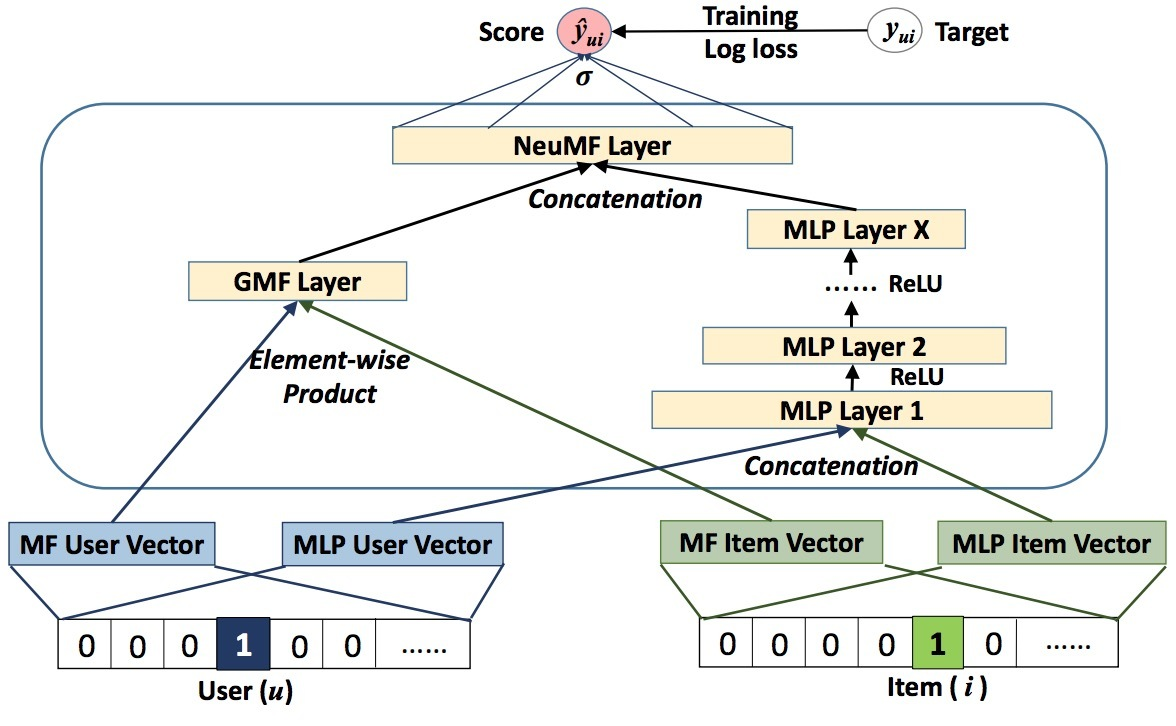$\phi^{GMF} = p_u^G \odot q_i^G \\\ \phi^{MLP} = a_L(W_L^T (a_{L-1} (... a_2(W_2^T \begin{bmatrix} p_u^M \\ q_i^M \end{bmatrix} + b_2)...)) + b_L) \\\ \hat{y}_{ui} = \sigma(h^T \begin{bmatrix} \phi^{GMF} \\ \phi^{MLP} \end{bmatrix}$

…(12)

### 3.4.1 预训练

$h \leftarrow \begin{bmatrix} \alpha h^{GMF} \\ (1-\alpha)h^{MLP} \end{bmatrix}$

…(13)

# 4.实验

• RQ1: 我们的NCF方法效果是否比state-of-art的隐式协同过滤方法效果要好?
• RQ2: 我们的最优化框架（负采样的log loss）是否适合推荐任务？
• RQ3: 更深layers的hidden units是否对学习user-item interaction数据更有用？

# 参考

https://www.comp.nus.edu.sg/~xiangnan/papers/ncf.pdf

# CTPN介绍

CTPN(Connectionist Text Proposal Network)由Zhi Tian等人提出。

# 总览

CTPN可以在卷积特征图（convolutional feature maps）中直接检测在精密尺度（fine-scale）的text proposals序列中的文本行（text line）。它开发了一个垂直锚点机制（vertical anchor mechanism），可以联合预测关于每个固定宽度proposal的位置（location）和文本/非文本分值（text/none-text score）。序列化的proposals被连接到一个RNN上，无缝地与卷积网络相接，产生一个end-to-end的训练模型。这使得CTPN可以探索丰富的图像文本信息，可以检测相当模糊的文本。CTPN可以在多尺度（multi-scale）和多语言文本（multi-language text）环境下可靠工作，无需进一步后序处理（post-processing）：这与自底向上的方法不同（它们通常需要多步后置过滤（post filtering））。在ICDAR 2013和2015 becnmarks上分别取得0.88和0.61的F-measure值，比最近的较好结果有很大的提升。CTPN的计算效率是0.14s/image，它使用了very deep VGG16 model.

# 一、介绍

## 1.1

CTPN的主要架构见图1.• 文本检测：
• 目标检测：

# 3.Connectionist Text Proposal Network

CTPN包括了三个主要部分：

• 在fine-scale proposals中检测文本
• 对text proposals进行recurrent连接（recurrent connectionist text proposals）
• 边缘细化（side-refinement）

## 3.1 Detecting Text in Fine-scale Proposals$v_c = (c_y - c_y^a)/h^a, v_h = log(h/h^a)$ …(1)

$v_c^* = (c_y^* - c_y^a)/h^a, v_h^* = log(h^*/h^a)$ …(2)

• $v={v_c, v_h}$和 $v^* = {v_c^*, v_h^*}$分别是相对预测坐标与ground true坐标。
• $c_y^a$和$h^a$是中心(y轴)和锚点的高度，它们可以从一个输入图片中被预计算好。
• $c_y$和h是预测的y轴坐标，$c_y^*$和$h^*$是ground truth坐标。因此，每个预测的text proposal具有一个size=hx16的边界，如图1(b)和图2(右)所示。通常，一个text proposal会大大小于有效可接受field（228x228）。

## 3.2 Recurrent Connectionist Text Proposals

$H_t = \phi(H_{t-1}, X_t), t=1,2,...,W$ …(3)

$H_t$的内部state被映射到下一个FC layer，以及output layer上用于计算第t个proposal的预测。因此，我们的与RNN layer集合的方式是优雅的，可以产生一个有效的模型，可以进行end-to-end训练，无需额外开销。RNN连接的高效性如图3所示。很明显，它减小了错误检测，同时，可以恢复许多缺失的text proposals（它们包含了非常弱的文本信息）。## 3.3 Side-refinement

fine-scale text proposals可以通过CTPN进行准确检测。文本行构建很简单，通过将那些text/no-text score > 0.7的连续的text proposals相连接即可。文本行的构建如下。首先，为一个proposal $B_i$定义一个邻居（$B_j$）：$B_j -> B_i$，其中：

• (i) $B_j$在水平距离上离$B_i$最近
• (ii) 该距离小于50 pixels
• (iii) 它们的垂直重叠(vertical overlap) > 0.7fine-scale detection和RNN连接可以预测在垂直方向上的累积位置。在水平方向上，图片被划分成一串16-pixel宽的proposals序列。当在两个水平侧（ horizontal sides）的text proposals不能准确被一个ground truth文本行区域覆盖时，或者一些side proposals被丢弃时（例如：具有一个较低的text score），这会导致一个不精准的定位，如图4所示。这种不准确在通用目标检测中不是很严格，但在文本检测中不能忽视，尤其是对于那些小尺度文本行或词。为了解决该问题，我们提供了一个side-refinement方法，可以准确地为每个anchor/proposal估计在水平左侧和水平右侧的offset（被称为side-anchor或side-proposal）。与y轴坐标的预测相似，我们计算了相对offset：

$o = (x_{side} - c_x^a) / w^a, o^{*} = (x_{side}^{*} - c_x^a) / w^a$…(4)

## 3.4 模型输出和Loss functions

CTPN有三个outputs，它们会一起连接到最后的FC layer上，如图1(a)所示。三个outputs同时预测：text/non-text scores(s)、垂直坐标(等式(2)中的$v={v_c, v_h}$ )、side-refinement offset (o)。我们探索了k个anchors来在conv5中的每个空间位置上预测它们，在各自的output layer上产生2k, 2k和k个参数。

$L(s_i, v_j, o_k) = \frac{1}{N_s} \sum_{i} L_s^{cl}(s_i, s_i^{*}) + \frac{\lambda_1}{N_v} \sum_j L_v^{re}(v_j, v_j^{*}) + \frac{\lambda_2}{N_o} \sum_k L_o^{re}(o_k, o_k^{*})$ ……(5)

## 3.5 训练和实现细节

CTPN通过标准的BP和SGD来进行end-to-end的训练。与RPN相似，训练样本是anchors，它们的位置可以通过在输入图片中的位置进行预计算得到，因而每个anchor的labels可以从相应的BT box计算得到。

Training Labels：对于text/none-text分类，会分配一个二分类label：positive（文本）、negative（非文本）anchor。它们通过计算BT bounding box（通过anchor位置进行划分）的IoU overlap得到。一个positive anchor被定义为：

• i. 一个具有一个与任意GB box有IoU>0.7的重合度(overlap)的anchor
• ii. 一个anchor具有一个与GT box的最高IoU重合度的anchor